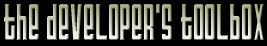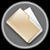This section of the archives stores flipcode's complete Developer Toolbox collection, featuring a variety of mini-articles and source code contributions from our readers.Minimize Recompilation When Using Inlines   Submitted byMost of my classes have inlined methods : Get/Set, operators, simple constructors, etc. For the sake of readability I'm in the habit of separating their implementation from the declaration of the class, by putting them in a .inl file (the MFC way). The .inl file is included at the end of the .h file, so apart from the aesthetic consideration it is exactly the same thing as coding the functions'bodies in the class itself : if a single inlined function is modified, every .cpp file including this class directly or not is going to be recompiled, which can take some time in a big project.

Considering that I develop most of the time in debug mode (and compile in release when everything seems OK), and that functions are by default not inlined at all in debug mode under Visual C++, there is a solution to minimize the recompilation problem : put the functions to be inlined in the .cpp file for a debug build, and in the .h file for a release build (so that they can really be inlined). Let's take an example to see how this works ; suppose I have the following class :

 ```//---------------------------------------------------------------------------// // VectMat.h file #ifndef _VECTMAT_H_ #define _VECTMAT_H_ #pragma onceclass CVect2D { public: // constructors inline CVect2D (void); inline CVect2D (const float fD); // x=y=fD inline CVect2D (const float fX, const float fY); // x=fX; y=fY [... etc ...] };#include "VectMat.inl" #endif // _VECTMAT_H_ //---------------------------------------------------------------------------// // VectMat.inl file #ifndef _VECTMAT_INL_ #define _VECTMAT_INL_inline CVect2D::CVect2D() // do nothing {}inline CVect2D::CVect2D(const float fD) // x=y=fD { m_fV[_X_] = m_fV[_Y_] = fD; }inline CVect2D::CVect2D(const float fX,const float fY) // x=fX; y=fY { m_fV[_X_] = fX; m_fV[_Y_] = fY; }[... etc ...]#endif // _VECTMAT_INL_ //---------------------------------------------------------------------------// // VectMat.cpp file #include "stdafx.h" #include "VectMat.h"[... implementation of the methods that are not inlined ...] ```

As stated before, any modification in VectMat.inl will cause the recompilation of every .cpp file that includes VectMat.h, and vectors are the kind of classes that are used in a lot of places. Now let's see the modified version :

 ```//---------------------------------------------------------------------------// // VectMat.h file #ifndef _VECTMAT_H_ #define _VECTMAT_H_ #pragma once#ifdef _DEBUG #define INLINE #else #define INLINE inline #endifclass CVect2D { public: // constructors INLINE CVect2D (void); INLINE CVect2D (const float fD); // x=y=fD INLINE CVect2D (const float fX, const float fY); // x=fX; y=fY [... etc ...] }; #ifndef _DEBUG #include "VectMat.inl" #endif #endif // _VECTMAT_H_ //---------------------------------------------------------------------------// // VectMat.inl file #ifndef _VECTMAT_INL_ #define _VECTMAT_INL_INLINE CVect2D::CVect2D() // do nothing {}INLINE CVect2D::CVect2D(const float fD) // x=y=fD { m_fV[_X_] = m_fV[_Y_] = fD; }INLINE CVect2D::CVect2D(const float fX,const float fY) // x=fX; y=fY { m_fV[_X_] = fX; m_fV[_Y_] = fY; }[... etc ...]#endif // _VECTMAT_INL_ //---------------------------------------------------------------------------// // VectMat.cpp file #include "stdafx.h" #include "VectMat.h" #ifdef _DEBUG #include "VectMat.inl" #endif[... implementation of the methods that are not inlined ...] ```

Now when compiling in debug mode, only VectMat.cpp is affected by VectMat.inl's changes.
Let's summarize the modifications (shown in red) :

* in the .h file :
- an INLINE constant is defined
- all occurrences of the 'inline' keyword are replaced by 'INLINE'
- VectMat.inl is only included for a release build

* in the .inl file :
- the 'inline' keywords are replaced by 'INLINE'

* in the .cpp file :
- VectMat.inl is included for a debug build (in addition to VectMat.h)

The zip file viewer built into the Developer Toolbox made use of the zlib library, as well as the zlibdll source additions.